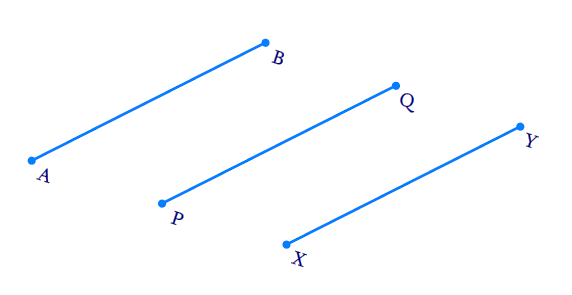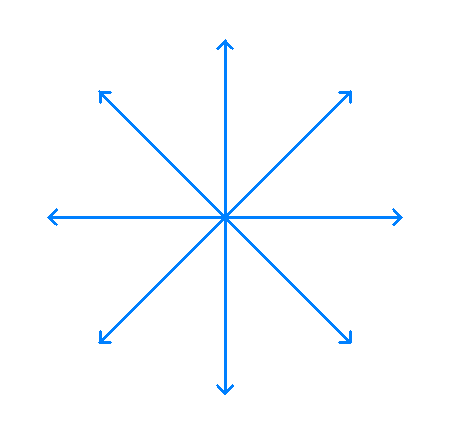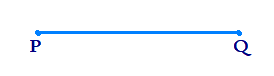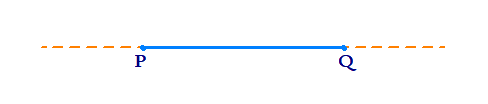# Ex.5.1 Q1 Introduction to Euclids Geometry Solution - NCERT Maths Class 9

## Question

Which of the following statements are true and which are false? Give reasons for your answers.

(i) Only one line can pass through a single point.

(ii) There are an infinite number of lines which pass through two distinct points.

(iii) A terminated line can be produced indefinitely on both the sides.

(iv) If two circles are equal, then their radii are equal.

(v) In figure 5.9  if

$$AB = PQ$$ and $$PQ = XY,$$

then $$AB = XY.$$Figure 5.9

Video Solution
Introduction To Euclids Geometry
Ex 5.1 | Question 1

## Text Solution

(i)  Reasoning:

We can draw infinite number of lines through a given point.

Steps:

FalseRelated problems:

1. What are concurrent lines?
2. How many chords can be drawn though center of the circle?

(ii) Reasoning:

According to Axiom 5.1: Given any two distinct points, there is a unique line that passes through them.

Steps:

False

We can draw only one line passing through two points.(iii)  Reasoning:

According to Postulate 2 :A terminated line can be produced indefinitely.

Steps:

True

We know that a straight line can be produced on both sides.(vi)  Reasoning:

According to Postulate 3: A circle can be drawn with any center and any radius.

Steps:

True

We know that circles are equal, means the circles are congruent. (Circles coinciding with each other). This means that circumferences are equal and so the radii of two circles are equal.

(v)  Reasoning:

Line segments whose corresponding lengths are equal are equal to one another.Steps:

True

By transitivity law, we know that, if $$a = b$$ and $$b = c$$ then $$a = c.$$

Here since $$AB = PQ$$and $$PQ = XY$$ then $$AB = XY$$ according to this law.

Let us consider $$AB =5 \;\rm{cm}$$, then $$PQ$$ will be $$5 \;\rm{cm}.$$

But $$PQ = XY$$ so $$XY$$ will also be $$5 \;\rm{cm}$$. $$AB = PQ = XY$$ which also implies $$AB = XY.$$

Learn from the best math teachers and top your exams

• Live one on one classroom and doubt clearing
• Practice worksheets in and after class for conceptual clarity
• Personalized curriculum to keep up with school